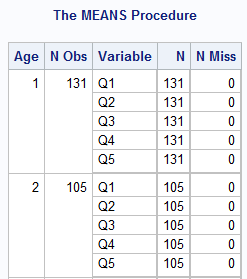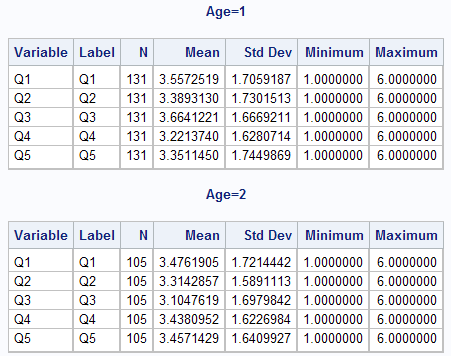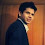# SAS : Detailed Explanation of Proc Means

PROC MEANS is one of the most common SAS procedure used for analyzing data. It is mainly used to calculate descriptive statistics such as mean, median, count, sum etc. It can also be used to calculate several other metrics such as percentiles, quartiles, standard deviation, variance and sample t-test.

## Uses of PROC MEANS

1. Analyze numeric or continuous variables
2. Analyze numeric variables by group(s)
3. Identifies outlier or extreme values
4. Hypothesis Testing with Sample T-test

## PROC MEANS Syntax

The syntax of PROC MEANS is shown below.

```PROC MEANS DATA=dataset-name ;
BY  variables;
CLASS variable(s) / ;
VAR variables;
OUTPUT OUT=SAS-data-set ;
RUN;
```

The explanation of statements of PROC MEANS is as follows :

• PROC MEANS - Calculate descriptive statistics for variables
• BY - Calculate separate statistics for each BY group
• CLASS - Group the analysis
• VAR - Numeric variables you want to analyze
• OUTPUT - Create an output data set
Dataset Description
The data includes seven variables and 499 observations. It comprises of survey responses from variables Q1 through Q5 and two demographics - Age and BU (Business Unit). The survey responses lie between 1 to 6.
To use the dataset in SAS, you can use PROC IMPORT to read data into SAS. See the code below -
out=test
dbms = xls;
run;

## Simple Example of PROC MEANS

In the DATA= option, you need to specify the dataset you want to use. In the VAR statement, you need to refer the numeric variables you want to analyze. You cannot refer character variables in the VAR statement.
Proc Means Data = test;
Var q1 - q5;
Run;

The output of PROC MEANS is shown in the image below.PROC MEANS OUTPUT

By default, PROC MEANS generates N, Mean, Standard Deviation, Minimum and Maximum statistics.

## Common Statistical Options of PROC MEANS

The most frequent statistical options used in PROC MEANS are listed below against their description.

Statistical Option Description
N Number of observations
NMISS Number of missing observations
MEAN Arithmetic average
STD Standard Deviation
MIN Minimum
MAX Maximum
SUM Sum of observations
MEDIAN 50th percentile
P1 1st percentile
P5 5th percentile
P10 10th percentile
P90 90th percentile
P95 95th percentile
P99 99th percentile
Q1 First Quartile
Q3 Third Quartile

Other Statistical Options

Statistical Option Description
VAR Variance
RANGE Range
USS Uncorr. sum of squares
CSS Corr. sum of squares
STDERR Standard Error
T Student’s t value for testing Ho: md = 0
PRT P-value associated with t-test above
SUMWGT Sum of the WEIGHT variable values
QRANGE Quartile range

## How to limit Descriptive Statistics

Suppose you want to see only two statistics - number of non-missing values and number of missing values.
Proc Means Data = test N NMISS;
Var q1 - q5 ;
Run;
N refers to number of non-missing values and NMISS implies number of missing values.PROC MEANS - Missing

Tips : Add NOLABELS option to delete Label column in the PROC MEAN table.
Proc Means data = test N NMISS NOLABELS;
Var q1 - q5;
Run;

## Group the analysis using PROC MEANS

Suppose you want to group or classify the analysis by Age. You can use the CLASS statement to accomplish this task. It is equivalent to GROUP BY in SQL.

Proc Means data = test N NMISS NOLABELS;
Class Age;
Var q1 - q5;
Run;Option Statement

You can use NONOBS option to delete N Obs column from the Proc Means table.
Proc Means data = test N NMISS NOLABELS NONOBS;
Class Age;
Var q1 - q5;
Run;

## How to use Format in Proc Means

First, you need to create an user defined format.
Proc Format;
Value Age
1 = 'Less than 25'
2 = '25-34'
3 = '35-43'
4 = '44-50'
5 = '51-59'
6 = '60 or more';
Run;
Add FORMAT statement to use user defined format in PROC MEANS.
Proc Means data = test N MEAN;
Class Age;
Format Age Age.;
Var q1 - q5;
Run;

## How to change Sorting Order

The DESCENDING option to the right of the slash in the first CLASS statement instructs PROC MEANS to analyze the data in DESCENDING order of the values of Age.
Proc Means Data = test;
Class Age / descending;
Var q1 - q5 ;
Run;
Instead of displaying the results in “sort order” of the values of the Classification Variable (s) you specified in the CLASS Statement, order the results by frequency order using the ORDER=FREQ option in the CLASS Statement.
Proc Means Data = test N;
Class Age / Order = FREQ;
Var q1 - q5 ;
Run;

You can order the results by user defined format of a variable specified in the CLASS statement using the ORDER=FORMATTED option in the CLASS Statement.
Proc Means data = test N MEAN;
Class Age / Order = formatted;
Format Age Age.;
Var q1 - q5;
Run;ORDER = FREQ Option

Note : If you specify CLASS statement without VAR statement, it classifies the analysis by all numeric variables in your data set.

## Grouping and Output in Separate Tables

Suppose you want to analyze variables Q1 - Q5 by variable AGE and want the output of each levels of AGE in separate tables. You can use BY statement to accomplish this task. See the example below-

Make sure you sort the data before using BY statement.
proc sort data= test;
by age;
run;
proc means data = test;
by age;
var q1 - q5 ;
run;

## Difference between CLASS and BY statement

The CLASS statement returns analysis for a grouping (classification) variable in a single table whereas BY statement returns the analysis for a grouping variable in separate tables. Another difference is CLASS statement does not require the classification variable to be pre-sorted whereas BY statement demands sorting.PROC MEANS Output

## Save output in a data set

You can use NOPRINT option to tell SAS not to print output in output window.
Proc Means data = test NOPRINT;
Class Age / Order = formatted;
Format Age Age.;
Var q1 - q5;
Output out = readin mean= median = /autoname;Run;
In the above code, readin is a data set in which output will be stored. The MEAN= MEDIAN= options tells SAS to generate mean and median in the output dataset. The AUTONAME Option automatically assigns unique variable names in the Output Data Set “holding” the statistics requested in the OUTPUT statement.
You can use AUTOLABEL option to automatically assigns unique label names in the Output Data Set “holding” the statistics requested in the OUTPUT statement.
Proc Means Data = test noprint;
Class Age ;
Var q1 q2;
Output out=F1 mean= / autoname autolabel;
Run;

You can specify variables for which you want summary statistics to be saved in a output data set.
Proc Means Data = test noprint;
Class Age ;
Var q1 q2;
Output out=F1 mean(q1)= median(q2)= / autoname;
Run;
You can give custom names to variables stored in a output data set.
Proc Means Data = test noprint;
Class Age;
Var q1 - q5 ;
Output out=F1 mean=_mean1-_mean5 median=_median1-_median5;Run;

DROP = , KEEP = option

We can use DROP and KEEP options to remove or keep some specific variables.
Proc Means Data = test noprint;
Class Age;
Var q1 - q5 ;
Output out=F1 (drop = _type_ _freq_) mean=_mean1-_mean5 median=_median1-_median5;
Run;

WHERE Statement

The WHERE statement is used to filter or subset data. In the code below, we are filtering on variable Q1 and telling SAS to keep only those observations in which value of Q1 is greater than 1.
Proc Means Data = test noprint;
Where Q1 > 1;Class Age;
Var q1 - q5 ;
Output out=F1(drop= _FREQ_) mean= median= / autoname;
Run;
Like WHERE statement, we can use WHERE= OPTION to filter data. See the following program -
Proc Means Data = test (Where=( Q1 > 1)) noprint;
Class Age;
Var q1 - q5 ;
Output out=F1(drop= _FREQ_) mean= median= / autoname;
Run;

## GROUPING on 2 (or more) Variables

When two ore more variables are included in the CLASS statement, PROC MEANS returns 3 levels of classification which is shown in the _TYPE_ variable. Suppose we are specifying variables AGE BU in the CLASS statement. SAS first returns mean and median of variables Q1-Q5 by BU. It is the first level of classification which can be filtered by using WHERE = ( _TYPE_ = 1). The same analysis by AGE is shown against _TYPE_ = 2. When _TYPE_ = 3, SAS returns analysis by both the variables AGE and BU.
Proc Means Data = test noprint;
Class Age BU;
Var q1 - q5 ;
Output out=F1 (where=(_type_=1) drop= AGE _FREQ_) mean= median= / autoname;
Output out=F2 (where=(_type_=2) drop= BU _FREQ_) mean= median= / autoname;
Output out=F3 (where=(_type_=3) drop= _FREQ_) mean= median= / autoname;
Run;
Using the NWAY option instructs PROC MEANS to output only observations with the highest value of _TYPE_ to the new data set it is creating.
Proc Means Data = test nway noprint;
Class Age;
Var q1 - q5 ;
Output out=F1 mean=_mean1-_mean5 median=_median1-_median5;
Run;
By default, PROC MEANS will analyze the numeric analysis variables at all possible combinations of the values of the classification variables. With the TYPES statement, only the analyses specified in it are carried out by PROC MEANS.
Proc Means Data = test noprint;
Class Age BU Q1;
Types()
Age * BU
Age * BU * Q1;
Var q1 - q5;
Output out=F1 mean=_mean1-_mean5 max=_median1-_median5;
Run;

DESCENDTYPES Option : Orders rows/observations in the output data set by descending value of _TYPE_.
Proc Means Data = test DESCENDTYPES noprint;
Class Age;
Var q1 - q5 ;
Output out=F1 mean=_mean1-_mean5 median=_median1-_median5;
Run;

## Multiple CLASS Statements

Multiple CLASS statement permit user control over how the levels of the classification variables are portrayed or written out to new data sets created by PROC MEANS. It means any one of the classification variable can be displayed in descending order.
Proc Means Data = test noprint;
Class Age / descending;
Class BU;
Var q1 - q5 ;
Output out=F1 mean=_mean1-_mean5 max=_median1-_median5;
Run;

## Identifying Extreme Values of Analysis Variables using the IDGROUP Option

proc means data=electric.electricity noprint nway;
class transformer;
var total_revenue ;
output out= F1
idgroup (max(total_revenue) out (total_revenue)=maxrev) idgroup (min(total_revenue) out (total_revenue)=minrev) sum= mean= /autoname;
run;

## Sample T-Test using PROC MEANS

With PROC MEANS, we can perform hypothesis testing using sample t-test.

Null Hypothesis - Population Mean of Q1 is equal to 0
Alternative Hypothesis - Population Mean of Q1 is not equal to 0.
proc means data = test t prt;
var Q1;
run;
The PRT option returns p-value which implies lowest level of significance at which we can reject null hypothesis. Since p-value is less than 0.05, we can reject the null hypothesis and concludes that mean is significantly different from zero.

## Difference between PROC MEANS and PROC FREQ

PROC MEANS is used to calculate summary statistics such as mean, count etc of numeric variables. It requires at least one numeric variable whereas Proc Freq does not have such limitation. In other words, if you have only one character variable to analyse, PROC FREQ is your friend and procedure to use.
Related Posts
ShareDeepanshu founded ListenData with a simple objective - Make analytics easy to understand and follow. He has over 10 years of experience in data science. During his tenure, he worked with global clients in various domains like Banking, Insurance, Private Equity, Telecom and HR.

22 Responses to "SAS : Detailed Explanation of Proc Means"
1.I need to create categories.
Example: Levels 1 - 6

I have the data set in SAS. Where to I start?

2.HI BALU HERE, MANY MANY THANKS FOR THIS PROC MEANS, PLZZZZZ EXPLAIN PROC FREQ TOO..

3.Awesome...keep going very informative

4.5.Hi Deepanshu,

I am using nolabels in sas 9.1 vmware but it is throwing errors

6.can you explain in Proc means what is n,mean,std,median,min,max.....

1.Did you miss description under 'Common Statistical Options' table?

7.Hi,
If I have a list of saleprice, but I need to get the mean of it (in ascending order) and categories under One variable FloorAreaCat. How do I write the code in Single Proc means step? (Note: I do not want to use Proc sort in this case)
Appreciate if can help.

8.Hi Deepanshu, I am using SAS on demand and am trying to import the test file however am getting this error.

Could you help me with the possible reasons for this?

9.ur listendata is very helpful sir..thank you so much..

10.Hi sir u gave good examples on proc means similarly I request u to upload examples on clinical domain

11.my i know what is p-value

12.It very nice tutorial short and precise.thank very much

13.Can you create a tutorial on Proc Report. Thanks

14.difference between these two where's??? performance???
Proc Means Data = test (Where=( Q1 > 1)) noprint;
Proc Means Data = test noprint;
Where Q1 > 1;Class Age;

1.See it in your log itself with realtime vs cpu time.

15.This comment has been removed by the author.

16.How can I do the IC of 95% confidency??? This way, don't work or I don't know interpret!
Proc means data=import1 ALPHA=0.05;
Var DMID1;
var DMID2;
var RFID1;
var RFID2;
output STDERR = stderr LCLM = lclm UCLM = uclm;
Run;

17.proc means data=electric.electricity noprint nway;
sir, where to find this data

18.hii
in proc means data step how can i get formats in statistics sum,mean,range etc....

19.20.Need elaborated theory on PROC MEANS

Next → ← Prev
Looks like you are using an ad blocker!

To continue reading you need to turnoff adblocker and refresh the page. We rely on advertising to help fund our site. Please whitelist us if you enjoy our content.